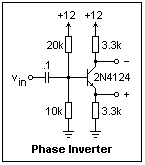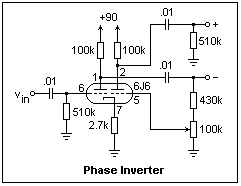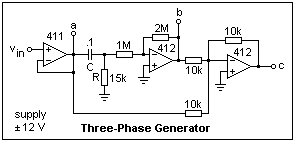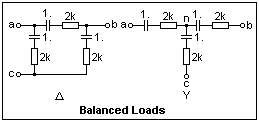## Phase Splitting

Phase splitting means the creation of a sinusoidal signal like a given one, but displaced in phase by a certain amount. A sinusoidal signal repeats a sequence of values as time passes, and the cycle is divided into 360 electrical degrees or 2π radians, like a circle. A rotating phasor is a graphic representation of these changes. The unit phasor exp(jωt) is said to have phase ωt in degrees or radians, where ω is the angular frequency 2πf radians per second. For 60 Hz, the angular frequency is the familar 377 rad/s. One signal is said to lead another if it goes through its phases a constant time before the other does, and this time can be specified as a fixed phase difference φ, which is more useful than a specification in terms of time. The phase lead is expressed as multiplication of the phasor by a complex factor exp(jφ). A signal is said to lag if it goes through its phases at a constant later time than the reference signal. Of course, a lag of 240° is the same as a lead of 120°, since the sinusoidal signals are periodic with period 360°.

Phase leads and lags are familiar from AC circuits. For a capacitor, some current must flow before the voltage can rise, so current leads voltage, which can be remembered by iCe. An inductor, on the other hand, requires a voltage to produce an increase in the current, so voltage leads current, or eLi. The phasor diagrams for series RC and RL circuits should be familiar. Since V = ZI, the inductive impedance jωL implies that V = ωLI will lead by 90°, since j = exp(j90°). Similarly, capacitative impedance 1/jωC implies that V will lag I, since -j = exp(-j90°).

### Phase Inversion

A special case of phase splitting is phase inversion, where a copy of a signal in antiphase is required. A phase inverter is required when one wishes to go from single-ended to double-ended operation. We have already seen how this can be done with a differential amplifier, where the signals taken from the two collectors are opposite in phase.

A transformer with a center-tapped secondary also produces phase inversion, since the signals at the ends of the secondary are in antiphase with respect to the center tap. This is a passive phase inverter, but a very practical one that was often used with vacuum tubes.

Two transistors or vacuum tubes can be used in push-pull. In this case, they are fed by opposite phases and biassed to be normally off. The tubes conduct on alternate half-cycles, each supplying half the output. This is called Class B operation, and is much more efficient than Class A operation where a tube or transistor must be biased so that its ouput can swing both ways. In Class B, no output current flows when there is no signal, saving a great waste of power and greatly increasing the efficiency of the amplifier. This has been discussed in more detail in another page.There are two convenient methods of phase inversion (in addition to the differential amplifier). The first depends on taking the outputs from resistors carrying the same current, which is controlled by the input signal, so that the phases are opposite. There is no voltage gain in this kind of phase inverter, so a preceding voltage amplification stage is usually required. A transistor phase inverter is shown at the left. The circuit is often called a paraphase amplifier. It should be biassed so that there is sufficient "headroom" for the two ouput signals. As the emitter voltage increases, the collector voltage decreases, and the limit is set when they collide, saturating the transistor. In this circuit, I have allowed about 5 V between collector and emitter when resting, which allows a peak-to-peak output of about this amount. The bias divider could probably be increased in value by a factor of 10 if a higher input impedance is required.The second method, more commonly used with vacuum tubes, uses a separate inverting amplifier to create the antiphase signal. There can be considerable gain in this kind of phase inverter, so a preamplifier is not required. Also, there are no headroom problems when a considerable output amplitude is desired. A circuit is shown at the right that uses a 6J6. Note that the input to the grid of the phase inverter is taken from the output of the other triode, in the grid circuit of the following tube, where a trimmer potentiometer can be used to adjust the ouputs to exact balance. The gain of this circuit is about 21, so the resistance below the slider is set at about 25k. The plate currents of both tubes flow through the same cathode resistor. Since the signal currents are opposite in phase, no signal current appears across the cathode resistor, and it need not be bypassed. This circuit produces a 30 V peak-to-peak ouput with an input of about 1.4 V. The octal 6N7 can be used in an identical circuit, and can feed a second 6N7 as a push-pull power output stage giving 10 W. This combination was often seen, in things like small PA systems. The same idea can be used with transistors. However, the availability of complementary transistors eliminates the need for phase inversion in most cases.

The phase inverters we have mentioned are all frequency-independent, both in phase shift and amplitude (within the bandwidth of the circuit), so that they act the same for all the frequency components of a complex signal. Phase inversion has no effect on the time-domain nature of the signal, and neither delays, advances nor distorts it.

### Polyphase Circuits

Power is usually delivered by sinusoidal voltages with a fixed phase difference. The most common is three-phase, where three conductors supply voltages differing in phase by 120°. This is the most efficient case, practically as efficient as DC transmission, which can deliver power steadily to a load without power-frequency fluctuations. It can also excite the rotating fields for efficient motors. The voltages measured between any two of the conductors are called line voltages, while the voltage measured between any one conductor and ground is called a phase voltage. The three phase voltages can be represented by three phasors of the same length, 120° apart, and the three line voltages by phasors connecting their tips. It is easily seen that the line voltages are √3 times the phase voltages. For example, if the phase voltage is 120V, then the line voltage is 208V. A system in which all the phases are in the same conditions is called balanced.

If you connect one side of three loads to the line conductors, and join the other sides of the loads to a common point, you have created a wye or Y load. On the other hand, if you connect the three loads across the three pairs of line conductors, you have created a delta or Δ load. Both work, but the voltages across the loads and the currents through them are different, of course. These connections are special cases of the more general star and mesh connections when there are an arbitrary number of line conductors. With balanced three-phase supply, the power supplied to the loads adds up to a constant, as for DC. In German, three-phase is called drehstrom, "rotating current," in contrast to gleichstrom (DC, "equal current") and wechselstrom (AC, "reversing current"), which emphasizes its special nature.

It is possible to supply two phases at 90° phase difference over three wires, one of them the neutral. This was the system favored by Tesla, and works very well for rotating machines, but is not as efficient as three-phase. It was originally used for power transmission, as at Niagara Falls, but this two-phase supply has been superseded by three-phase in all cases. American house supply is actually two-phase, where the phase difference is 180°. This Edison three-wire system was originally used for DC supply. Edison's claim that AC was dangerous ruled out any higher supply voltage for business reasons, so house supply is still only 120 V, though effectively distributed at 240 V with the three-wire system. Three-phase home service at a line voltage of 240 would be much more efficient, and probably safer if only line-to-line connections were made.If two-phase is necessary, the circuit at the left provides a signal leading the input by 90°. It is, of course, just an integrator with transfer function 1/jωCR. The phase shift does not depend on the frequency, but the ratio of the ouput and input amplitudes does. This circuit is designed to give equal amplitudes for 60 Hz. The 1M resistor is added so that the ouput will not drift if there is a small DC component in the input, perhaps from leakage. When the phase shifter is turned on, you may see a small notch in the ouput that will gradually disappear. The DC gain of the circuit is 37. Observe the relation of input and output using a Lissajous figure. It ought to produce a circle in an XY display. Vary the frequency to see what happens. The circle becomes an ellipse, but its axes remain vertical and horizontal, showing that the phase shift is constant at 90°.Three-phase is produced by the circuit shown at the right. Any op-amp can abe used, but 411's and 412's are shown. Here, both the phase shift and the amplitude are affected by the frequency, so the values of R and C must be chosen for the desired frequency. This RC circuit produces a phase shift of 60° when f = 1 / 2π√3 RC; about 60 Hz for this circuit. It is supplied by a buffer amplifier, which provides phase "a." The shifted signal is then multiplied by -2 to restore its amplitude and invert it, producing phase "b." Phases "a" and "b" are added and inverted by the third amplifier, and the result is phase "c." By careful adjustment of the gains and frequency, the balance can be made quite perfect, but it will be found good enough without any adjustments except for perhaps a slight change of frequency.

Check the phase and amplitude relations of the three phases with the oscilloscope. It is possible to display all three phases on my scope, using one phase as the trigger, but I did not take the trouble to connect a third input, and simply compared phase "a" separately with phases "b" and "c." It is well to note a restriction of the oscilloscope--the inputs must be referenced to the chassis ground of the oscilloscope, which is also, presumably, the ground of your breadboard supply. It cannot be used to measure a floating voltage directly, such as a line-to-line voltage in this case.

The DMM does not share this drawback of the oscilloscope, and we can use it to measure floating voltages. If we are using 60 Hz, the AC scales will work excellently, giving rms values. (The usual DMM measures the average absolute value and multiplies by 1.11, assuming a sine wave, which is OK here). Set the phase voltages at about 5.00 V, and measure the line voltages, which should be about 8.66 V. Unless you are very lucky, they will not be exactly equal, but close enough to show the general result. Connect three 10k resistors as a Y load, and measure the voltage at the common node. I found 0.058 V when the phase voltage was 5 V, pretty good balance. This generator of a three-phase signal can be used to model three-phase circuits, and to study many of their properties, such as the relation of loads and line currents, harmonics and effects of imbalance. It is easier to work with low voltages and mVA rather than with high voltages and kVA!To illustrate the use of the three-phase generator, consider the balanced loads shown at the left. They can be drawn to look more like a Δ or a Y, but this is not convenient here. Three equal RC branches are connected in delta on the left, and in wye on the right. The node "n" will be at ground potential if the system is exactly balanced. Measurements can be made of the line voltages ab, ac and bc, and of the line currents a, b and c. Use the ACV and ACmA scales of the DMM for the measurements. Putting the DMM in one line will unbalance the system a little, but not seriously. Let's consider the measurement of the power supplied to the load in the two cases. As with normal single-phase circuits, the power cannot be found by measurements of the magnitudes of voltages and currents, but phase information must also be included somehow.

If the load is purely resistive, then the power in each branch of the wye connection is VpIp, and the total power is 3VpIp = √3VLIL, since the phase voltage Vp = VL/√3. For the delta connection, the total power will be 3VLIp = √3VLIL, since the phase current Ip = IL/√3. In any case, then, the total power in a balanced load is √3VLIL, and can be found from the line voltages and currents alone. The factors of √3 come from the phase relations, of course. If the load is reactive, then it has a power factor pf, and the average power is the volt-amperes √3VLIL times pf.

In the example, the impedance of the RC branch at 60 Hz is 2.0 + j2.65 kΩ = 3.32 /_-52.96°, and the power factor is cos (-52.96°) = 0.602. Incidentally, 60 Hz is used so that the DMM will read correctly, since its AC scales are most accurate at this frequency. When the line-to-line voltage was 8.75 V, I found the line current in the delta connection to be 4.62 mA. It's easy to calculate the power by finding the phase current 8.75 V / 3.32 k = 2.64 mA, and then the power by (2.64)2 x 2 k = 13.89 mW, so the total average power is 41.68 mW. From what we have said above, this should agree with √3 x 8.75 x 4.62 x 0.602 = 42.15 mW, which it does, closely enough. The reader can repeat the calculation for the Y load, noticing that the phase voltage is VL/√3. For a line voltage of 8.67 V, I measured a line current of 1.51 mA. The total power delivered to the load in this case was 13.56 mW. If you follow all this, you should be able to make power measurements on balanced three-phase systems with ease.

Note that the wye and delta loads made from the same branches are by no means equivalent, giving different line currents. It is possible to design a wye load equivalent to a given delta load, and vice-versa. This wye-delta transformation is often useful in circuit analysis, and is easily found in a good circuits text, or in the AC Essentials page in this website, under Technology.